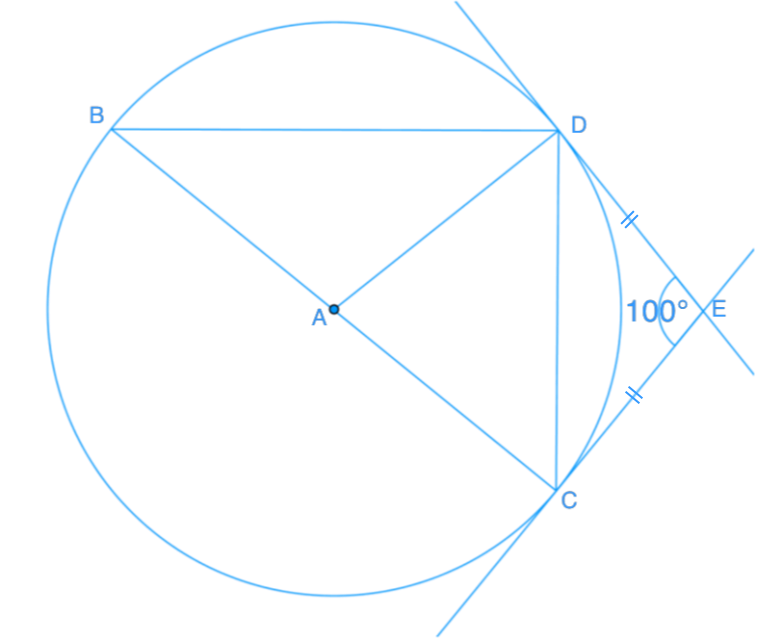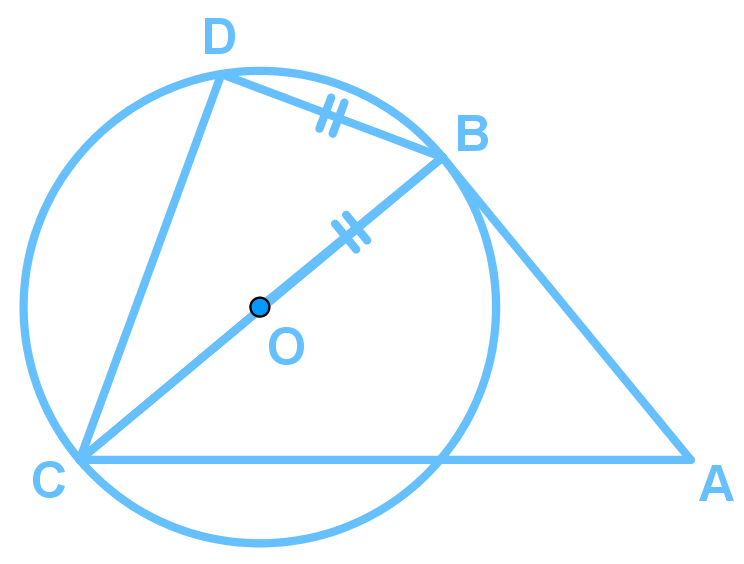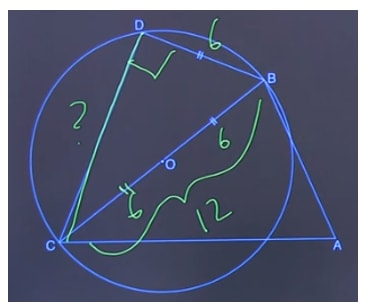# Tangent properties

0/2
##### Introduction
###### Lessons
1. What is a chord?
2. What is a tangent?
1. What are the properties of a tangent?
0/10
##### Examples
###### Lessons

1.Find.
1. $\angle$DCE
2. $\angle$CBD
3. $\angle$BAD
2. In the diagram below, BC is a diameter, and AB is tangent to the circle at point B. OB = 6 cm, and AB = 8 cm.1. Find the length of the diameter.
2. Justify if $\triangle$CBD is a right angle.
3. Find the length of the chord DC.
4. Find $\angle$ABC.
5. Find the length of AC.
6. If there is a straight line connecting point D and point O, what type of triangle is $\triangle$BOD?
3. The furthest the hammer can travel in a hammer throw game is when the hammer travels along a tangent. If a hammer lands 62 m away from the athlete, how far did the hammer travel along a tangent? (Hint: The hammer is 1.2 m in length.)
###### Free to Join!
StudyPug is a learning help platform covering math and science from grade 4 all the way to second year university. Our video tutorials, unlimited practice problems, and step-by-step explanations provide you or your child with all the help you need to master concepts. On top of that, it's fun - with achievements, customizable avatars, and awards to keep you motivated.
• #### Easily See Your ProgressWe track the progress you've made on a topic so you know what you've done. From the course view you can easily see what topics have what and the progress you've made on them. Fill the rings to completely master that section or mouse over the icon to see more details.
• #### Make Use of Our Learning Aids###### Practice Accuracy

See how well your practice sessions are going over time.

Stay on track with our daily recommendations.

• #### Earn Achievements as You LearnMake the most of your time as you use StudyPug to help you achieve your goals. Earn fun little badges the more you watch, practice, and use our service.
• #### Create and Customize Your AvatarPlay with our fun little avatar builder to create and customize your own avatar on StudyPug. Choose your face, eye colour, hair colour and style, and background. Unlock more options the more you use StudyPug.
###### Topic Notes
A tangent to a circle must form a perpendicular line from the point of tangency to the center of the circle. In this lesson, we will learn how to use this property, along with others such as, chord properties, to solve questions.

## What is a tangent of a circle

When you have a circle, a tangent is perpendicular to its radius. It touches (intersects) the circle at only one point and looks like a line that sits just outside the circle's circumference. The fact that it is perpendicular will come in useful in our calculations as we can then make use the Pythagorean theorem.

## How to find the tangent of a circle

In the questions you'll have to answer in this lesson, you'll either be given the tangent or you'll have to look for it. Some tangent properties that you should keep in mind to help you solve problems include:

1) A tangent is perpendicular to the radius at the point of tangency.

2) Tangent segments to an external point of a circle are equal.

3) The angle between a tangent and a chord is equal to the inscribed angle on the opposite side of that chord.

You'll see these properties in use in the practice problems coming up when dealing with a (or several) tangent line on a circle.

## What is a chord in a circle

In a circle, a chord is a line that has both endpoints lying on the circle. A chord has several properties and some of them are:

1) If two chords' lengths are equal in a circle, they are equidistant from the center.

2) The longest chord in a circle is the diameter and it passes through the center of a circle.

3) A perpendicular bisector of a chord passes through the center of a circle.

## Example problems

Question 1:

Find angle CBD

Solution:

First, look for useful information from the graph:

Triangle CDE is an isosceles triangle.

Angle CED is 100°. Therefore angle CDE and angle DCE = 40°

Point A is the center of the circle.

Point D and point C are point of tangency to the circle. Line AD and line AC are perpendicular to the tangent line. Therefore, Angle ACE = 90°.

Angle ACD = Angle ACE – Angle DCE = 90° - 40° = 50°

Angle CDB is an inscribed angle of the diameter. So, Angle CDB = 90°.

angle CBD = 180° - Angle ACD - Angle CDB = 180° - 50° - 90° = 40°

Question 2:

In the diagram below, BC is a diameter, and AB is tangent to the circle at point B. OB = 6 cm, and AB = 8 cm.

Find the length of the chord DC.

Solution:

OB is the radius = 6 cm. So, the diameter BC = 12 cm

OB = DB – 6 cm

Angle CDB = 90° because it is an inscribed angle of the diameterAngle CDB = 90° because of inscribed angle of the diameter

Using the Pythagorean theorem:

$c^{2} = a^{2} + b^{2}$

$12^{2} = 6^{2} +b^{2}$

$\sqrt{12^{2} - 6^{2}} = b$

$b = \sqrt{108}$

$b = 10.39cm$

Question 3

Find the length of AC

Solution:

AB is tangent to the circle. So angle ABC = 90°

OB is the radius = 6 cm. So, the diameter BC = 12 cm

AB = 8cm

Using the Pythagorean theorem:

$c^{2} = a^{2} + b^{2}$

$c = \sqrt{12^{2} + 8^{2}}$

$c = \sqrt{80}$

$c = 8.94cm$

Check out this online calculator to help you figure out the lengths of the tangents of a circle. Ready to build off on this lesson? Learn about the arcs of a circle, area of a circle, and central and inscribed angles.